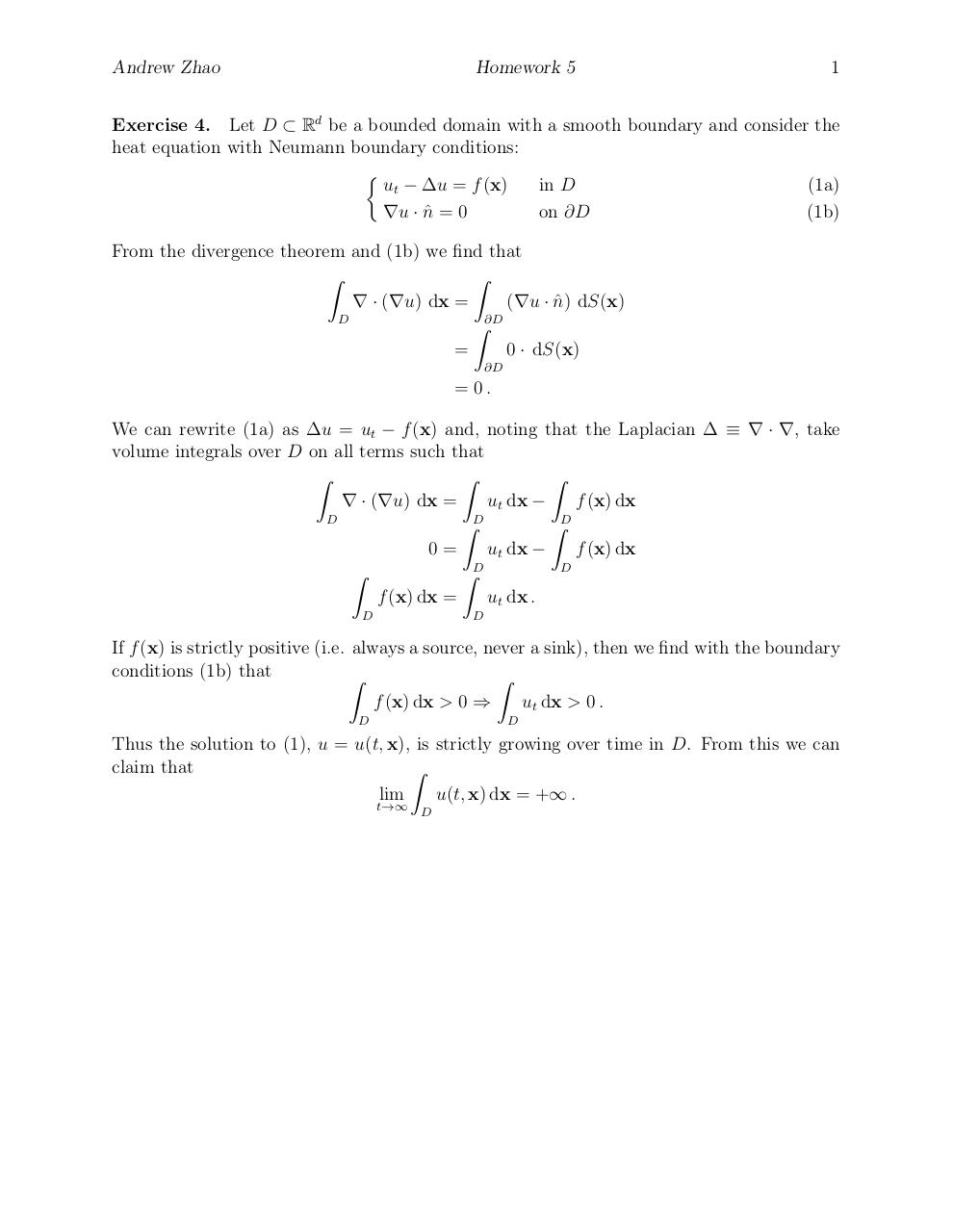# 462 5 4 .pdf

### File information

Original filename: 462_5_4.pdf

This PDF 1.5 document has been generated by TeX / MiKTeX pdfTeX-1.40.15, and has been sent on pdf-archive.com on 23/10/2015 at 23:30, from IP address 69.143.x.x. The current document download page has been viewed 380 times.
File size: 106 KB (1 page).
Privacy: public file

462_5_4.pdf (PDF, 106 KB)

### Document preview

Andrew Zhao

Homework 5

1

Exercise 4. Let D ⊂ Rd be a bounded domain with a smooth boundary and consider the
heat equation with Neumann boundary conditions:

ut − ∆u = f (x)
in D
(1a)
∇u · n
ˆ=0
on ∂D
(1b)
From the divergence theorem and (1b) we find that
Z
Z
∇ · (∇u) dx =
(∇u · n
ˆ ) dS(x)
D
Z∂D
=
0 · dS(x)
∂D

= 0.
We can rewrite (1a) as ∆u = ut − f (x) and, noting that the Laplacian ∆ ≡ ∇ · ∇, take
volume integrals over D on all terms such that
Z
Z
Z
∇ · (∇u) dx =
ut dx −
f (x) dx
D
D
D
Z
Z
f (x) dx
ut dx −
0=
D
Z
ZD
f (x) dx =
ut dx .
D

D

If f (x) is strictly positive (i.e. always a source, never a sink), then we find with the boundary
conditions (1b) that
Z
Z
f (x) dx &gt; 0 ⇒

ut dx &gt; 0 .
D

D

Thus the solution to (1), u = u(t, x), is strictly growing over time in D. From this we can
claim that
Z
u(t, x) dx = +∞ .
lim
t→∞

D#### HTML Code

Copy the following HTML code to share your document on a Website or Blog

#### QR Code### Related keywords